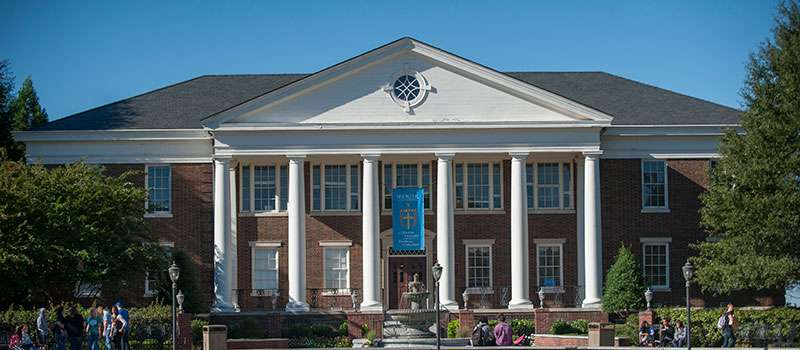Mathematics Course Descriptions – Shorter UniversityMAT 0990: Foundations of Mathematics (0)

A course designed to prepare students for college algebra. Admission is by assignment. This course carries three semester hours institutional load credit, but it does not satisfy degree requirements.

MAT 1060: Mathematics for Liberal Arts (3)

This course will explore major contributions of mathematics to humanity. Beginning with a brief history of mathematics and mathematical systems, the study will include units on symbolic logic, mathematical functions and graphs, problem solving using basic algebraic equations, and descriptive statistics.

MAT 1110: College Algebra (3)

Properties of real numbers, order and absolute value, complex numbers, scientific notation, factoring polynomials, linear and quadratic equations, systems of equations, linear inequalities, and graphing.

Prerequisite: MAT 0990 or exemption.

MAT 1120: Precalculus Math (3)

The algebra of exponential, logarithmic, and trigonometric functions, including identities, inverse trigonometric functions, and right triangle trigonometry.

Prerequisite: MAT 1110 or departmental consent.

MAT 1150: Mathematics for ECE/MGE: Arithmetic and Algebra (3)

This course covers measurement and geometry, along with data analysis and probability. The course also emphasizes a thorough understanding of the mathematical concepts covered, at the level necessary to teach the concepts to elementary and middle school students.

MAT 1160: Mathematics for ECE/MGE: Measurement and Analysis (3)

This course covers measurement and geometry, along with data analysis and probability. The course also emphasizes a thorough understanding of the mathematical concepts covered, at the level necessary to teach the concepts to elementary and middle school students.

MAT 2040: Introduction to Programming (3)

Utilizes a high level object oriented language to introduce basic computer programming constructs. Topics include computer organization, programming structure and syntax, graphics, program flow, decision structures, simulations and algorithmic development. (Cross-listed with CIS 2040)

Prerequisite: MAT 1110 or departmental consent.

MAT 2080: Mathematics for Middle Grades (3)

Fraction arithmetic, number theory, geometry of three dimensional shapes, statistics and probability are covered along with other middle grades mathematics content, with an emphasis on a deeper understanding of these concepts and common student difficulties at the middle school level.

MAT 2120: Introduction to Discrete Mathematics (3)

An introduction to the methods of discrete mathematics. Topics covered will include elementary set theory, symbolic logic, mathematical induction, combinatorics, recurrence relations, and graph theory.

Prerequisite: MAT 1110.

MAT 2610: Calculus I (4)

A brief review of algebra and trigonometry; coordinate systems, analytical geometry, the derivative using the definition, limits, continuity, techniques of differentiation; Mean value theorem and its application, applications of differentiation to extreme value problems, curve sketching and related rates problems, the integral and its properties, applications of the integral for finding area under a curve, antiderivatives, and Fundamental Theorem of Calculus.

Prerequisite: MAT 1120

MAT 2620: Calculus II (4)

Further study of the integral, volume of a solid of revolution, length of a curve, area of a surface of revolution, work, moments, and centroids. Applications of differential and integral calculus to improper integrals, infinite series, polynomial approximations of functions, Taylor’s Theorem, conics, and polar coordinates.

Prerequisite: MAT 2610

MAT 3020: Euclidean Geometry (3)

This course will cover concepts in Euclidean geometry, using an axiomatic system with an emphasis on formal proofs.

MAT 3180: Introduction to Statistics (3)

Frequency distributions; their graphic and tabular representations; measures of central tendency, of dispersion and of correlation; sampling; elementary probability theory.

Prerequisites: MAT 1110 or departmental consent.

MAT 3380: Calculus III (4)

Applications of differential and integral calculus to vector-valued functions, partial derivative, multiple integrals, vector field analysis, line and surface integrals, Jacobian transformations, Green, Stokes, and Gauss Theorems.

Prerequisite: MAT 2620

MAT 3450: Introduction to Problem Solving with Computers (3)

Problems from calculus and real world applications will be approached through the use of the FORTRAN programming language and various software packages such as DERIVE, MATHEMATICA, MATHCAD, and MAPLE.

Prerequisites: MAT 2610, 2620.

MAT 3990: Special Topics (1-4)

Variable subject content. (Repeatable Course)

MAT 4010: Linear Algebra (3)

Covers fields, systems of linear equations, matrices, determinants, vector spaces, and linear transformations.

Prerequisite: MAT 2620 or departmental consent.

MAT 4020: Modern Algebra (3)

An introduction to the study of abstract algebraic systems, covering the elementary theory of groups, rings, and fields.

Prerequisite: MAT 2620.

MAT 4030: Differential Equations (3)

An introductory course in ordinary differential equations with applications. Topics covered include first and second order differential equations, power series solutions, Laplace transforms, linear systems, and numerical methods.

Prerequisite: MAT 2620.

MAT 4050: History of Mathematics (3)

This course covers a variety of topics related to the development of mathematical concepts and conventions, from ancient mathematics to modern times.

MAT 4200: Real Analysis (3)

This course covers sequences and series, convergence, divergence, functional limits, open, closed, and compact sets, and additional analysis topics. The course emphasizes a thorough understanding of developing and writing proofs of basic Real Analysis theorems.